# Kunneth formula for homology

(Redirected from Kunneth formula)

## Statement

Suppose$X$ and$Y$ are topological spaces. We then have the following relation for the homology groups of$X$,$Y$, and the product space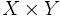$X \times Y$.

For any$n \ge 0$ and any module$M$ over a principal ideal domain$R$ for coefficients, we have: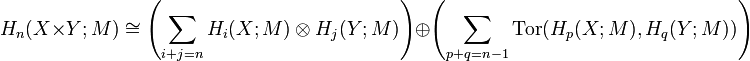$H_n(X \times Y;M) \cong \left(\sum_{i + j = n} H_i(X;M) \otimes H_j(Y;M)\right) \oplus \left(\sum_{p + q = n-1} \operatorname{Tor}(H_p(X;M),H_q(Y;M))\right)$

Here,$\operatorname{Tor}$ is torsion of modules over the ring$R$.

## Particular cases

### Case of free modules

If all the homology groups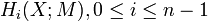$H_i(X;M), 0 \le i \le n - 1$ are free (or more generally torsion-free) modules over$R$, and/or all the homology groups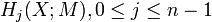$H_j(X;M), 0 \le j \le n - 1$, are free (or more generally torsion-free) modules over$R$, then all the torsion part vanishes and we get: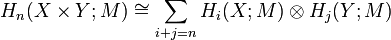$H_n(X \times Y;M) \cong \sum_{i + j = n} H_i(X;M) \otimes H_j(Y;M)$

In particular, if all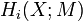$H_i(X;M)$ and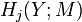$H_j(Y;M)$ are free modules over$R$ and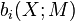$b_i(X;M)$ and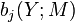$b_j(Y;M)$ denote the respective free ranks, and all these are finite, we obtain that: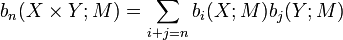$b_n(X \times Y;M) = \sum_{i + j = n} b_i(X;M)b_j(Y;M)$

Note that if$R$ is a field, then the above holds.

### Impact for ranks even in case of torsion

When$R$ is a principal ideal domain and all the homologies are finitely generated modules over$R$, we can consider the rank as the rank of the torsion-free part of the homology modules. If$b_i(X;M)$ denotes the free rank of the torsion-free part of$H_i(X;M)$, we get:$b_n(X \times Y;M) = \sum_{i + j = n} b_i(X;M)b_j(Y;M)$

Note that this applies even if the homology modules have torsion.

In the special case that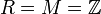$R = M = \mathbb{Z}$, the numbers$b_i$ are called Betti numbers, and we get: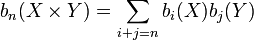$b_n(X \times Y) = \sum_{i+j=n}b_i(X)b_j(Y)$

In particular, this yields that Poincare polynomial of product is product of Poincare polynomials.

## Facts used

The Kunneth formula combines the Kunneth theorem and the Eilenberg-Zilber theorem.

## Proof

Fill this in later